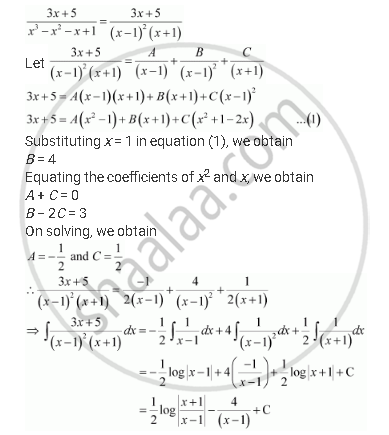Share
Notifications

View all notifications
Books Shortlist
Your shortlist is empty

# Solution for Integrate the Rational Functions (3x + 5)/(X^3 - X^2 - X + 1) - CBSE (Science) Class 12 - Mathematics

Login
Create free account

Forgot password?
ConceptMethods of Integration - Integration Using Partial Fractions

#### Question

Integrate the rational functions (3x + 5)/(x^3 - x^2 - x + 1)

#### SolutionIs there an error in this question or solution?

#### Video TutorialsVIEW ALL 

Solution Integrate the Rational Functions (3x + 5)/(X^3 - X^2 - X + 1) Concept: Methods of Integration - Integration Using Partial Fractions.
S